International
Tables for
Crystallography
Volume B
Reciprocal space
Edited by U. Shmueli

International Tables for Crystallography (2006). Vol. B, ch. 1.4, pp. 102-104   | 1 | 2 |

## Section 1.4.3. Structure-factor tables

U. Shmuelia

### 1.4.3. Structure-factor tables

| top | pdf |

#### 1.4.3.1. Some general remarks

| top | pdf |

This section is a revised version of the structure-factor tables contained in Sections 4.5 through 4.7 of Volume I (IT I, 1952). As in the previous edition, it is intended to present a comprehensive list of explicit expressions for the real and the imaginary parts of the trigonometric structure factor, for all the 17 plane groups and the 230 space groups, and for the hkl subsets for which the trigonometric structure factor assumes different functional forms. The tables given here are also confined to the case of general Wyckoff positions (IT I, 1952). However, the expressions are presented in a much more concise symbolic form and are amenable to computation just like the explicit trigonometric expressions in Volume I (IT I, 1952). The present tabulation is based on equations (1.4.2.19)and (1.4.2.20),i.e. the numerical coefficients in A and B which appear in Tables A1.4.3.1–A1.4.3.7in Appendix 1.4.3are appropriate to space-group-specific structure-factor formulae. The functional form of A and B is, however, the same when applied to Fourier summations (see Section 1.4.2.3).

#### 1.4.3.2. Preparation of the structure-factor tables

| top | pdf |

The lists of the coordinates of the general equivalent positions, presented in IT A (1983), as well as in earlier editions of the Tables, are sufficient for the expansion of the summations in (1.4.2.19)and (1.4.2.20)and the simplification of the resulting expressions can be performed using straightforward algebra and trigonometry (see, e.g., IT I, 1952). As mentioned above, the preparation of the present structure-factor tables has been automated and its stages can be summarized as follows:

 (i) Generation of the coordinates of the general positions, starting from a computer-adapted space-group symbol (Shmueli, 1984). (ii) Formation of the scalar products, appearing in (1.4.2.19)and (1.4.2.20), and their separation into components depending on the rotation and translation parts of the space-group operations: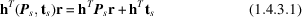for the space groups which are not associated with a unique axis; the left-hand side of (1.4.3.1)is separated into contributions of the relevant plane group and unique axis for the remaining space groups. (iii) Analysis of the translation-dependent parts of the scalar products and automatic determination of all the parities of hkl for which A and B must be computed and simplified. (iv) Expansion of equations (1.4.2.19)and (1.4.2.20)and their reduction to trigonometric expressions comparable to those given in the structure-factor tables in Volume I of IT (1952). (v) Representation of the results in terms of a small number of building blocks, of which the expressions were found to be composed. These representations are described in Section 1.4.3.3.

All the stages outlined above were carried out with suitably designed computer programs, written in numerically and symbolically oriented languages. A brief summary of the underlying algorithms is presented in Appendix 1.4.1. The computer-adapted space-group symbols used in these computations are described in Section A1.4.2.2and presented in Table A1.4.2.1.

#### 1.4.3.3. Symbolic representation of A and B

| top | pdf |

We shall first discuss the symbols for the space groups that are not associated with a unique axis. These comprise the triclinic, orthorhombic and cubic space groups. The symbols are also used for the seven rhombohedral space groups which are referred to rhombohedral axes (IT I, 1952; IT A, 1983).

The abbreviation of triple products of trigonometric functions such as, e.g., denoting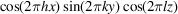by csc, is well known (IT I, 1952), and can be conveniently used in representing A and B for triclinic and orthorhombic space groups. However, the simplified expressions for A and B in space groups of higher symmetry also possess a high degree of regularity, as is apparent from an examination of the structure-factor tables in Volume I (IT I, 1952), and as confirmed by the preparation of the present tables. An example, illustrating this for the cubic system, is given below.

The trigonometric structure factor for the space group(No. 200) is given by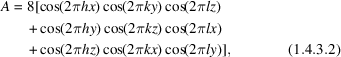and the sum of the above nine-function block and the following one: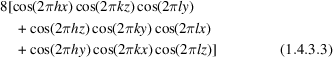is the trigonometric structure factor for the space group(No. 221, IT I, 1952, IT A, 1983). It is obvious that the only difference between the nine-function blocks in (1.4.3.2)and (1.4.3.3)is that the permutation of the coordinates xyz is cyclic or even in (1.4.3.2), while it is non-cyclic or odd in (1.4.3.3).

It was observed during the generation of the present tables that the expressions for A and B for all the cubic space groups, and all the relevant hkl subsets, can be represented in terms of such even' and odd' nine-function blocks. Moreover, it was found that the order of the trigonometric functions in each such block remains the same in each of its three terms (triple products). This is not surprising since each of the above space groups contains threefold axes of rotation along  and related directions, and such permutations of xyz for fixed hkl (or vice versa) are expected. It was therefore possible to introduce two permutation operators and represent A and B in terms of the following two basic blocks: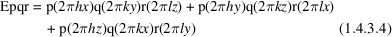and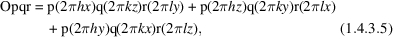where each of p, q and r can be a sine or a cosine, and appears at the same position in each of the three terms of a block. The capital prefixes E and O were chosen to represent even and odd permutations of the coordinates xyz, respectively.

For example, the trigonometric structure factor for the space group(No. 205, IT I, 1952, IT A, 1983) can now be tabulated as follows: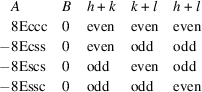(cf. Table A1.4.3.7), where the sines and cosines are abbreviated by s and c, respectively. It is interesting to note that the only maximal non-isomorphic subgroup of, not containing a threefold axis, is the orthorhombic Pbca (see IT A, 1983, p. 621), and this group–subgroup relationship is reflected in the functional forms of the trigonometric structure factors; the representation of A and B for Pbca is in fact analogous to that of, including the parities of hkl and the corresponding forms of the triple products, except that the prefix E – associated with the threefold rotation – is absent from Pbca. The expression for A for the space group[the sum of (1.4.3.2)and (1.4.3.3)] now simply reads: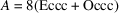.

As pointed out above, the permutation operators also apply to rhombohedral space groups that are referred to rhombohedral axes (Table A1.4.3.6), and the corresponding expressions for R3 andbear the same relationship to those for P1 and(Table A1.4.3.2), respectively, as that shown above for the relatedand Pbca.

When in any given standard space-group setting one of the coordinate axes is parallel to a unique axis, the point-group rotation matrices can be partitioned intoand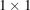diagonal blocks, the former corresponding to an operation of the plane group resulting from the projection of the space group down the unique axis. If, for example, the unique axis is parallel to c, we can decompose the scalar product in (1.4.2.19)and (1.4.2.20)as follows: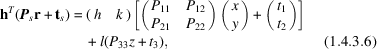where the first scalar product on the right-hand side of (1.4.3.6)contains the contribution of a plane group and the second product is the contribution of the unique axis itself. The above decomposition often leads to a convenient factorization of A and B, and is applicable to monoclinic, tetragonal and hexagonal families, the latter including rhombohedral space groups that are referred to hexagonal axes.

The symbols used in Tables A1.4.3.3, A1.4.3.5and A1.4.3.6are based on such decompositions. In those few cases where explicit expressions must be given we make use of the convention of replacing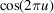by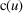and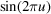by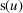. For example,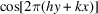etc. is given as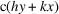etc. The symbols are defined below.

 Monoclinic space groups (Table A1.4.3.3)The following symbols are used in this system: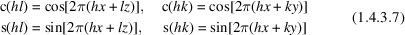so that any expression for A or B in the monoclinic system has the form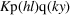or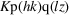for the second or first setting, respectively, where p and q can each be a sine or a cosine and K is a numerical constant. Tetragonal space groups (Table A1.4.3.5)The most frequently occurring expressions in the summations for A and B in this system are of the form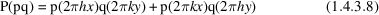and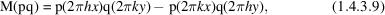where p and q can each be a sine or a cosine. These are typical contributions related to square plane groups. Trigonal and hexagonal space groups (Table A1.4.3.6)The contributions of plane hexagonal space groups to the first term in (1.4.3.6)are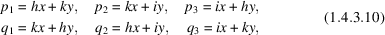where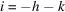(IT I, 1952). The symbols which represent the frequently occurring expressions in this family, and given in terms of (1.4.3.10), are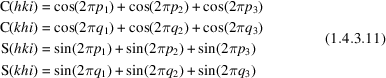and these quite often appear as the following sums and differences: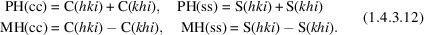The symbols defined in this section are briefly redefined in the appropriate tables, which also contain the conditions for vanishing symbols.

#### 1.4.3.4. Arrangement of the tables

| top | pdf |

The expressions for A and B are usually presented in terms of the short symbols defined above for all the representations of the plane groups and space groups given in Volume A (IT A, 1983), and are fully consistent with the unit-cell choices and space-group origins employed in that volume. The tables are arranged by crystal families and the expressions appear in the order of the appearance of the corresponding plane and space groups in the space-group tables in IT A (1983).

The main items in a table entry, not necessarily in the following order, are: (i) the conventional space-group number, (ii) the short Hermann–Mauguin space-group symbol, (iii) brief remarks on the choice of the space-group origin and setting, where appropriate, (iv) the real (A) and imaginary (B) parts of the trigonometric structure factor, and (v) the parity of the hkl subset to which the expressions for A and B pertain. Full space-group symbols are given in the monoclinic system only, since they are indispensable for the recognition of the settings and glide planes appearing in the various representations of monoclinic space groups given in IT A (1983).

### References

International Tables for Crystallography (1983). Vol. A. Space-group symmetry, edited by Th. Hahn. Dordrecht: Reidel. (Present distributor Kluwer Academic Publishers, Dordrecht.)
International Tables for X-ray Crystallography (1952). Vol. I. Symmetry groups, edited by N. F. M. Henry & K. Lonsdale. Birmingham: Kynoch Press.
Shmueli, U. (1984). Space-group algorithms. I. The space group and its symmetry elements. Acta Cryst. A40, 559–567.